﻿ 基于有限差分法的多介质高温作业服设计的优化数学模型 An Optimization Mathematical Model for Design of Multi-Medium High Temperature Operation Suit Based on Finite Difference Method

Vol. 08  No. 02 ( 2019 ), Article ID: 29028 , 16 pages
10.12677/AAM.2019.82038

An Optimization Mathematical Model for Design of Multi-Medium High Temperature Operation Suit Based on Finite Difference Method

Baochen Sun, Yijun Wu*

College of Mathematical Sciences, Tianjin Normal University, Tianjin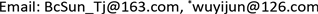Received: Jan. 31st, 2019; accepted: Feb. 19th, 2019; published: Feb. 26th, 2019ABSTRACT

This paper mainly studies the one-dimensional temperature distribution and thickness optimization of a typical high temperature operation special suit. Firstly, the finite difference method is used to discretize the continuous heat transfer model, and the undetermined parameters are estimated by using the constrained nonlinear programming, and the internal temperature distribution of the garment in 75 degrees Celsius high temperature environment is simulated. Secondly, by reducing the constraint conditions to simplify the single-objective planning problem, the optimal value is 17.6 mm by using the Fibonacci method, the Golden section method, the dichotomy method and the two-interpolation method. Finally, an algorithm for effectively shortening the interval length to solve the feasible boundary points is proposed, and a simple complete layered sequence method is used to solve the multi-objective programming model, and the optimal thickness of the II and IV layers is 19.2 mm and 6.4 mm.

Keywords:Unsteady Heat Conduction Model, Multi-Objective Programming, Constrained Nonlinear Programming, Finite Difference, Simple and Complete Hierarchical Sequence Method1. 引言Table 1. Parameter values for special garment materials

2. 问题分析

3. 模型的建立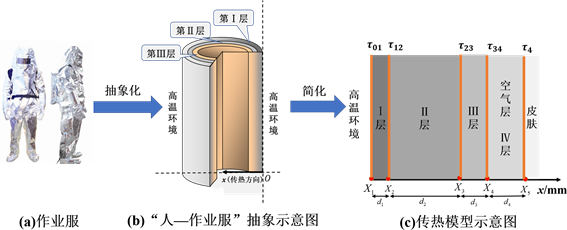Figure 1. Model Abstraction diagram

3.1. “模型一”的建立Table 2. Main heat transfer mode of fabric material and air layerFigure 2. Diagram of the Thin bar

$\Delta Q=cm\Delta u$ ,

$\Delta Q=c\rho S\Delta x\left(u\left(x,t+\Delta t\right)-u\left(x,t\right)\right)$ ,

$\Delta t\to {0}_{+}$ 时，

$\Delta Q=c\rho S\Delta x\frac{\partial u}{\partial t}\left(x,t\right)\Delta t$ .(1)

$\Delta Q=-\lambda \frac{\partial u}{\partial {e}_{n}}\left(x,t\right)S\Delta t$ ,. (2)

${Q}_{in}=-\lambda \frac{\partial u}{\partial x}\left(x,t\right)S\Delta t$ ; (3)

${Q}_{out}=-\lambda \frac{\partial u}{\partial x}\left(x+\Delta x,t\right)S\Delta t$ . (4)

$\Delta Q={Q}_{in}-{Q}_{out}=-\lambda \left(\frac{\partial u}{\partial x}\left(x,t\right)-\frac{\partial u}{\partial x}\left(x+\Delta x,t\right)\right)S\Delta t$ . (5)

$c\rho \text{ }\Delta x\frac{\partial u}{\partial t}\left(x,t\right)=\lambda \left(\frac{\partial u}{\partial x}\left(x+\Delta x,t\right)-\frac{\partial u}{\partial x}\left(x,t\right)\right)$ ,

$\frac{\partial u}{\partial t}\left(x,t\right)=\frac{\lambda }{c\rho }\frac{\left(\frac{\partial u}{\partial x}\left(x+\Delta x,t\right)-\frac{\partial u}{\partial x}\left(x,t\right)\right)}{\Delta x}\triangleq a\frac{\left(\frac{\partial u}{\partial x}\left(x+\Delta x,t\right)-\frac{\partial u}{\partial x}\left(x,t\right)\right)}{\Delta x}$ ,

$\frac{\partial u}{\partial t}\left(x,t\right)=a\frac{{\partial }^{2}u}{\partial {x}^{2}}\left(x,t\right)$ .

$\frac{\partial u}{\partial t}={a}_{i}\frac{{\partial }^{2}u}{\partial {x}^{2}},\left(x,t\right)\in \left({X}_{i},{X}_{i+1}\right)×\left(0,T\right],{a}_{i}=\frac{{\lambda }_{i}}{{c}_{i}\Delta {\rho }_{i}}\left(i=1,2,3,4\right)$ ,

(A1)初始条件：假设进入高温环境时，人体与作业服已达到稳定状态，作业服温度分布的初始值为假人温度37℃，即 $u\left(x,0\right)=37\left(x\in \left[{X}_{1},{X}_{5}\right]\right)$

(A2)边界条件：牛顿冷却定律表明，当物体表面与周围存在温度差时，单位时间从单位面积散失的热量与温度差成正比  。在传热系统左边界 $x={X}_{1}$ 和右边界 $x={X}_{5}$ 分别应用之，结合式(2)得到两个第三类边界条件，即

$-{\lambda }_{1}\frac{\partial u}{\partial x}\left({X}_{1},t\right)={h}_{1}\left({u}_{env}-u\left({X}_{1},t\right)\right)$ ,

${\lambda }_{4}\frac{\partial u}{\partial x}\left({X}_{5},t\right)={h}_{2}\left(u\left({X}_{5},t\right)-37\right)$ ,

(A3)交界条件：假设材料间接触良好，忽略接触热阻，在交界面上温度与热流密度连续，结合式(2)，可得

$\left\{\begin{array}{l}u\left({X}_{i}-0,t\right)=u\left({X}_{i}+0,t\right)\\ {\lambda }_{i}\frac{\partial u}{\partial x}\left({X}_{i+1}-0,t\right)={\lambda }_{i+1}\frac{\partial u}{\partial x}\left({X}_{i+1}+0,t\right)\end{array},i=1,2,3$ .

$\left\{\begin{array}{l}\frac{\partial u}{\partial t}={a}_{i}\frac{{\partial }^{2}u}{\partial {x}^{2}},\left(x,t\right)\in \left({X}_{i},{X}_{i+1}\right)×\left(0,T\right),{a}_{i}=\frac{{\lambda }_{i}}{{c}_{i}\Delta {\rho }_{i}}\left(i=1,2,3,4\right)\\ \left(初值条件\right)u\left(x,0\right)=37\left(x\in \left[{X}_{1},{X}_{5}\right]\right)\\ \left(边界条件\right)\left\{\begin{array}{l}-{\lambda }_{1}\frac{\partial u}{\partial x}\left({X}_{1},t\right)={h}_{1}\left({u}_{env}-u\left({X}_{1},t\right)\right)\\ {\lambda }_{4}\frac{\partial u}{\partial x}\left({X}_{5},t\right)={h}_{2}\left(u\left({X}_{5},t\right)-37\right)\end{array}\\ \left(交界条件\right)\left\{\begin{array}{l}u\left({X}_{i}-0,t\right)=u\left({X}_{i}+0,t\right)\\ {\lambda }_{i}\frac{\partial u}{\partial x}\left({X}_{i+1}-0,t\right)={\lambda }_{i+1}\frac{\partial u}{\partial x}\left({X}_{i+1}+0,t\right)\end{array}\left(i=1,2,3\right)\end{array}$ .

3.2. “模型二”、“模型三”的建立

${\delta }_{j}=\left\{\begin{array}{l}0,j\notin A\\ 1,j\in A\end{array}$ ,

$v={p}_{1}{\delta }_{1}{d}_{1}+{p}_{2}{\delta }_{2}{d}_{2}+\cdots +{p}_{n}{\delta }_{n}{d}_{n}$ ,

$w={\rho }_{1}{\delta }_{1}{d}_{1}+{\rho }_{2}{\delta }_{2}{d}_{2}+\cdots +{\rho }_{n}{\delta }_{n}{d}_{n}+{\rho }_{n+1}{\delta }_{n+1}{d}_{n+1}$ ,

$h={\delta }_{1}{d}_{1}+{\delta }_{2}{d}_{2}+\cdots +{\delta }_{n}{d}_{n}+{\delta }_{n+1}{d}_{n+1}$ .Table 3. Objective function of establishing multi-objective programming model

$\begin{array}{l}\mathrm{min}{p}_{2}{d}_{2}\\ \mathrm{min}{\rho }_{2}{d}_{2}\\ \mathrm{min}{d}_{2}\\ \text{s}.\text{t}.\left\{\begin{array}{l}u\left({X}_{5},3600\right)\le 47\\ u\left({X}_{5},3300\right)\le 44\\ 0.6\le {d}_{2}\le 25\end{array}\end{array}$ $⇔$ $\begin{array}{l}\mathrm{min}{d}_{2}\\ \text{s}.\text{t}.\left\{\begin{array}{l}u\left({X}_{5},3600\right)\le 47\\ u\left({X}_{5},3300\right)\le 44\\ 0.6\le {d}_{2}\le 25\end{array}\end{array}$

$\begin{array}{l}\mathrm{min}{p}_{2}{d}_{2}\\ \mathrm{min}{\rho }_{2}{d}_{2}+{\rho }_{4}{d}_{4}\\ \mathrm{min}{d}_{2}+{d}_{4}\\ \text{s}.\text{t}.\text{\hspace{0.17em}}\left\{\begin{array}{l}u\left({X}_{5},3600\right)\le 47\\ u\left({X}_{5},3300\right)\le 44\\ 0.6\le {d}_{2}\le 25\\ 0.6\le {d}_{4}\le 6.4\end{array}\end{array}$ $⇔$ $\begin{array}{l}\mathrm{min}{d}_{2}\\ \mathrm{min}{\rho }_{2}{d}_{2}+{\rho }_{4}{d}_{4}\\ \mathrm{min}{d}_{2}+{d}_{4}\\ \text{s}.\text{t}.\text{\hspace{0.17em}}\left\{\begin{array}{l}u\left({X}_{5},3600\right)\le 47\\ u\left({X}_{5},3300\right)\le 44\\ 0.6\le {d}_{2}\le 25\\ 0.6\le {d}_{4}\le 6.4\end{array}\end{array}$

4. 模型的求解

4.1. 问题1求解Table 4. Stability and truncation error of several differential format solutions, of which r = Δ t Δ x 2

$\frac{{u}_{j}^{k}-{u}_{j}^{k-1}}{\Delta t}=\frac{a}{2}\left(\frac{{u}_{j+1}^{k}-2{u}_{j}^{k}+{u}_{j-1}^{k}}{\Delta {x}^{2}}+\frac{{u}_{j+1}^{k-1}-2{u}_{j}^{k-1}+{u}_{j-1}^{k-1}}{\Delta {x}^{2}}\right)$

$\left\{\begin{array}{l}-{a}_{i}ru\left({x}_{j-1},{t}_{k}\right)+\left(2{a}_{i}r+2\right)u\left({x}_{j},{t}_{k}\right)-{a}_{i}ru\left({x}_{j+1},{t}_{k}\right)={a}_{i}ru\left({x}_{j-1},{t}_{k-1}\right)+\left(2-2{a}_{i}r\right)u\left({x}_{j},{t}_{k-1}\right)+{a}_{i}ru\left({x}_{j+1},{t}_{k-1}\right)\\ \left(初值条件\right)u\left({x}_{j},0\right)=37\\ \left(边界条件\right)\left\{\begin{array}{l}\left(\frac{{\lambda }_{1}}{\Delta x}+{h}_{1}\right)u\left({x}_{1},{t}_{k}\right)-\frac{{\lambda }_{1}}{\Delta x}u\left({x}_{2},{t}_{k}\right)={u}_{env}{h}_{1}\\ \left(\frac{{\lambda }_{4}}{\Delta x}+{h}_{2}\right)u\left({x}_{m},{t}_{k}\right)-\frac{{\lambda }_{4}}{\Delta x}u\left({x}_{m-1},{t}_{k}\right)=37{h}_{2}\end{array}\\ \left(交界条件\right)-\frac{{\lambda }_{i}}{\Delta x}u\left({x}_{{B}_{i}-1},{t}_{k}\right)+\frac{{\lambda }_{i}+{\lambda }_{i+1}}{\Delta x}u\left({x}_{{B}_{i}},{t}_{k}\right)-\frac{{\lambda }_{i+1}}{\Delta x}u\left({x}_{{B}_{i}+1},{t}_{k}\right)=0\left(i=1,2,3\right)\end{array}$

$\left(\begin{array}{ccc}\begin{array}{ccc}\frac{{\lambda }_{1}}{\Delta x}+{h}_{1}& -\frac{{\lambda }_{1}}{\Delta x}& \\ -{a}_{1}r& 2{a}_{1}r+2& -{a}_{1}r\\ & -{a}_{1}r& 2{a}_{1}r+2\end{array}& \begin{array}{ccc}& & \\ & & \\ & & \end{array}& \begin{array}{ccc}& & \\ & & \\ & & \end{array}\\ \begin{array}{ccc}& & \ddots \\ & & \\ & & \end{array}& \begin{array}{ccc}\ddots & & \\ -\frac{{\lambda }_{1}}{\Delta x}& \frac{{\lambda }_{1}+{\lambda }_{2}}{\Delta x}& -\frac{{\lambda }_{2}}{\Delta x}\\ & \ddots & \ddots \end{array}& \begin{array}{ccc}& & \\ & & \\ & & \end{array}\\ \begin{array}{ccc}& & \\ & & \\ & & \end{array}& \begin{array}{ccc}& & \\ & & \\ & & \end{array}& \begin{array}{ccc}2{a}_{4}r+2& -{a}_{4}r& \\ -{a}_{4}r& 2{a}_{4}r+2& -{a}_{4}r\\ & -\frac{{\lambda }_{4}}{\Delta x}& \frac{{\lambda }_{4}}{\Delta x}+{h}_{2}\end{array}\end{array}\right)$

$\left(\begin{array}{c}\begin{array}{c}37\\ 37\end{array}\\ \begin{array}{c}⋮\\ 37\end{array}\end{array}\right)\stackrel{解方程}{\to }\left(\begin{array}{c}\begin{array}{c}u\left({x}_{1},{t}_{1}\right)\\ u\left({x}_{2},{t}_{1}\right)\end{array}\\ \begin{array}{c}⋮\\ u\left({x}_{m},{t}_{1}\right)\end{array}\end{array}\right)\stackrel{解方程}{\to }\cdots \stackrel{解方程}{\to }\left(\begin{array}{c}\begin{array}{c}u\left({x}_{1},{t}_{n}\right)\\ u\left({x}_{2},{t}_{n}\right)\end{array}\\ \begin{array}{c}⋮\\ u\left({x}_{m},{t}_{n}\right)\end{array}\end{array}\right)$

${e}_{r}\left({h}_{1},{h}_{2}\right)$ 为在75℃高温环境下工作90分钟后人体皮肤外侧温度相对误差向量，本文首先对未知参数 ${h}_{1}$${h}_{2}$ 有约束非线性规划问题Table 5. Optimal solution h 1 and h 2 obtained by constrained nonlinear programming(a) (b)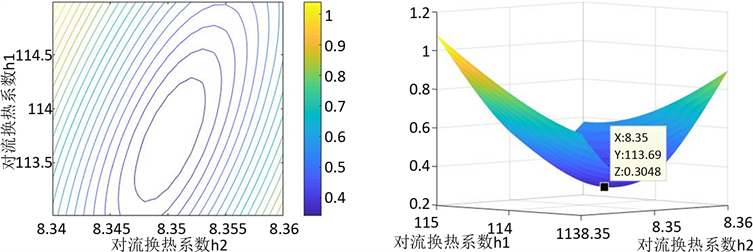(c) (d)

Figure 3. Contour map obtained from the traversal search and ${h}_{1}-{h}_{2}-{‖{e}_{r}\left({h}_{1},{h}_{2}\right)‖}_{2}$ figureTable 6. Where Δ x units are in millimeters and Δ t units are in secondsFigure 4. (a) Calculation results and errors of the lateral temperature of human skin. (b) Calculation results of temperature distribution

4.2. 问题2求解

${u}_{3600}\left({d}_{2}\right)\le {u}_{3600}\left(0.6\right)=45.101124$

$\begin{array}{l}\mathrm{min}{d}_{2}\\ \text{s}\text{.t}\text{.}\text{\hspace{0.17em}}\left\{\begin{array}{l}u\left({X}_{5},3300\right)\le 44\\ 0.6\le {d}_{2}\le 25\end{array}\end{array}$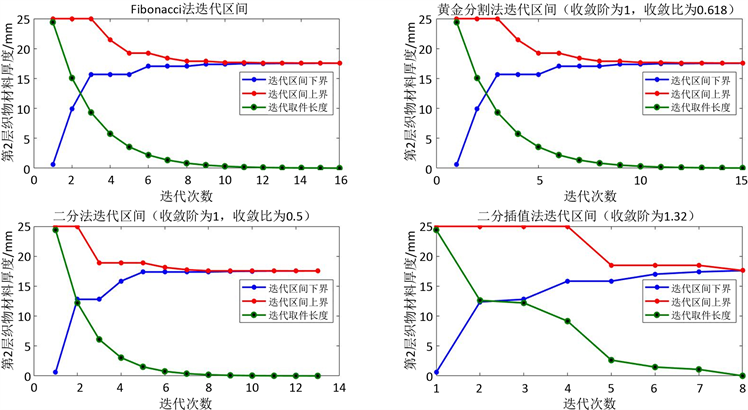Figure 5. Diagram of iterative interval of 4 search methods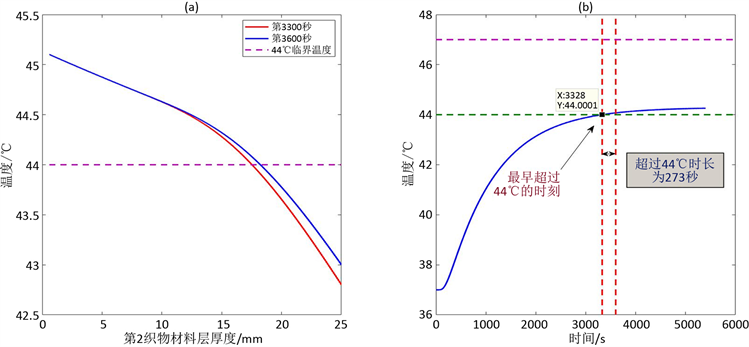Figure 6. (a) The thickness of the II fabric material layer is ${d}_{2}$ at 0.01 for 3,300 seconds and 3,600 seconds. (b) The temperature change of the lateral temperature of the human skin within 60 minutes of the operation of the II fabric material layer thickness ${d}_{2}=17.55\text{\hspace{0.17em}}\text{mm}$

4.3. 问题3求解

$\begin{array}{l}\mathrm{min}{d}_{2}\\ \mathrm{min}{\rho }_{2}{d}_{2}+{\rho }_{4}{d}_{4}\\ \mathrm{min}{d}_{2}+{d}_{4}\\ \text{s}.\text{t}.\text{\hspace{0.17em}}\left\{\begin{array}{l}u\left({X}_{5},3600\right)\le 47\\ u\left({X}_{5},3300\right)\le 44\\ 0.6\le {d}_{2}\le 25\\ 0.6\le {d}_{4}\le 6.4\end{array}\end{array}$

${u}_{1800}\left({d}_{2},{d}_{4}\right)\le {u}_{1800}\left(0.6,0.6\right)=58.553266$ (6)

${u}_{1500}\left({d}_{2},{d}_{4}\right)\le {u}_{1500}\left(0.6,0.6\right)=58.553266$ (7)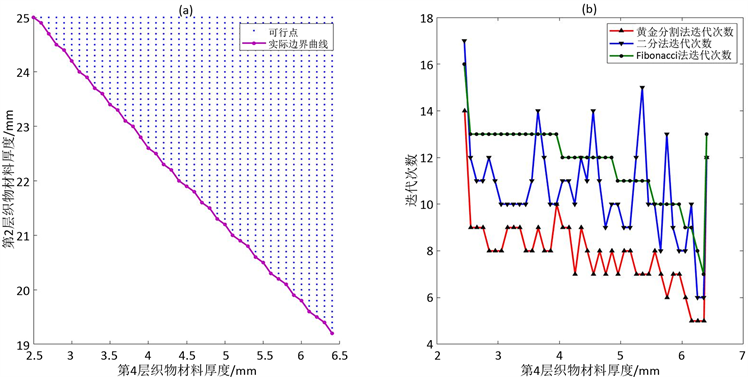Figure 7. (a) Initial feasible domain; (b) Number of iterations of new algorithms in the application of different one-dimensional search methods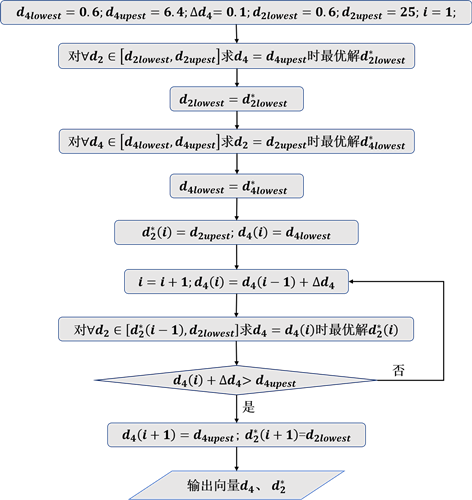Figure 8. Algorithm flowchart

An Optimization Mathematical Model for Design of Multi-Medium High Temperature Operation Suit Based on Finite Difference Method[J]. 应用数学进展, 2019, 08(02): 334-349. https://doi.org/10.12677/AAM.2019.82038

1. 1. 卢琳珍. 多层热防护服装的热传递模型及参数最优决定[D]: [硕士学位论文]. 杭州: 浙江理工大学, 2018.

2. 2. 潘斌. 热防护服装热传递数学建模及参数决定反问题[D]: [硕士学位论文]. 杭州: 浙江理工大学, 2017.

3. 3. 赵军. 矫直辊工作寿命的研究[J]. 机械工程师, 2013(5): 142-144.

4. 4. 杨世铭, 陶文铨. 传热学[M]. 第4版. 北京: 高等教育出版社, 2006.

5. 5. 毛君, 朱煜, 陈洪月, 袁智, 宋振铎. 采煤机摇臂齿轮固热耦合分析与齿廓修形[J]. 煤炭学报, 2017, 42(6): 1598-1606.

6. 6. 宋淑红, 王双虎. 带间断扩散系数热传导方程的新型自适应数值解法[J]. 应用数学学报, 2010, 33(5): 942-960.

7. 7. 张文生. 科学计算中的偏微分方程有限差分法[M]. 北京: 高等教育出版社, 2006: 40-44, 235-245.

8. 8. 陆金甫, 关治. 偏微分方程数值解[M]. 第3版. 北京: 清华大学出版社, 2016: 96.

9. 9. 王健, 赵国生, 宋一兵, 等. MATLAB数值计算基础与示例教程[M]. 北京: 机械工业出版社, 2018: 255-258.

10. 10. 戴嘉尊, 邱建贤. 微分方程数值解法[M]. 第2版. 南京: 东南大学出版社, 2012: 57-61.

11. 11. 解可新, 韩健, 林友联. 最优化方法[M]. 第2版. 天津: 天津大学出版社, 2004: 12-23, 241-245.